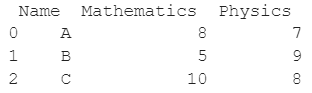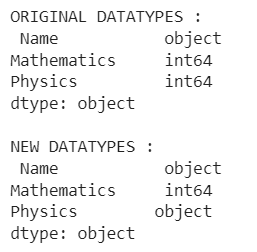# How To Convert Columns To String In A Pandas DataFrame?

A DataFrame is the primary data structure of the Pandas library and is commonly used for storing and working with tabular data. A common operation that could be performed on such data is to convert columns to string in order to modify existing information.

To start working with Pandas, we first need to import it in Python code :

### Python 3 Code :

``import pandas as pd``

## Running Example

Let us understand this operation with the help of an example. Consider the following DataFrame containing 3 students with names ‘A’, ‘B’ and ‘C’ and their corresponding marks (out of 10) for two subjects, Mathematics and Physics.Code snippet for generating the above DataFrame :

### Python 3 Code :

``````import pandas as pd

# Dictionary for our data
data = {'Name' : ['A', 'B', 'C'], 'Mathematics' : [8, 5, 10], 'Physics' : [7, 9, 8]}

# DataFrame for the dictionary
df = pd.DataFrame(data)

# Printing the DataFrame
print(df)``````

Here, data is a dictionary we created to initialize the DataFrame. For this, we use the DataFrame() function of the Pandas library which takes the dictionary as an argument and returns the required DataFrame.

Now let us say that for some reason, we want to convert the column with label Physics to string type.

Pay attention to the fact that the type of the Physics column changed from int64 to object, a standard type for string.

On checking the original and new datatypes after conversion, the resulting output will look as follows :Let us look at different ways of performing this operation on a given DataFrame :

## Convert Columns To String In A Pandas DataFrame Using the astype() function

In this method, we use the astype() function to convert a column to a string in a given Pandas DataFrame.

Let the column of interest be the one with the label as Physics here. The .astype() function takes the datatype of interest as a parameter, here str, and returns the converted column object.

The conversion is not in place so reassignment of the returned object is required.

Let us take a look at the code and corresponding output for this method. Pay attention to the fact that the type of the Physics column changed from int64 to object, a standard type for string.

### Python 3 Code :

``````import pandas as pd

# Dictionary for our data
data = {'Name' : ['A', 'B', 'C'], 'Mathematics' : [8, 5, 10], 'Physics' : [7, 9, 8]}

# DataFrame for the dictionary
df = pd.DataFrame(data)

# Print the original datatypes
print("ORIGINAL DATATYPES :\n",df.dtypes)

# Convert the column Physics to string
df['Physics'] = df['Physics'].astype(str)

# Printing the new datatypes
print("\nNEW DATATYPES :\n", df.dtypes)``````

Output :## Convert Columns To String In A Pandas DataFrame Using the astype() function with the values property

In this method, we use the .astype() function to convert a column to a string in a given Pandas DataFrame.

Let the column of interest be the one with the label as Physics here. The values function returns the values contained in the desired column.

The .astype() function takes the datatype of interest as a parameter, here str, and returns the converted column object. The conversion is not in place so reassignment of the returned object is required.

Let us take a look at the code and corresponding output for this method.

Pay attention to the fact that the type of the Physics column changed from int64 to object, a standard type for string.

### Python 3 Code :

``````import pandas as pd

# Dictionary for our data
data = {'Name' : ['A', 'B', 'C'], 'Mathematics' : [8, 5, 10], 'Physics' : [7, 9, 8]}

# DataFrame for the dictionary
df = pd.DataFrame(data)

# Print the original datatypes
print("ORIGINAL DATATYPES :\n",df.dtypes)

# Convert the column Physics to string
df['Physics'] = df['Physics'].values.astype(str)

# Printing the new datatypes
print("\nNEW DATATYPES :\n", df.dtypes)``````

Output :## Convert Columns To String In A Pandas DataFrame Using the map() function

In this method, we use the map() function to convert a column to a string in a given Pandas DataFrame. Let the column of interest be the one with the label as Physics here.

The map() function takes the datatype of interest as a parameter, here str, and returns the converted column object. The conversion is not in place so reassignment of the returned object is required.

Let us take a look at the code and corresponding output for this method.

Pay attention to the fact that the type of the Physics column changed from int64 to object, a standard type for string.

### Python 3 Code :

``````import pandas as pd

# Dictionary for our data
data = {'Name' : ['A', 'B', 'C'], 'Mathematics' : [8, 5, 10], 'Physics' : [7, 9, 8]}

# DataFrame for the dictionary
df = pd.DataFrame(data)

# Print the original datatypes
print("ORIGINAL DATATYPES :\n",df.dtypes)

# Convert the column Physics to string
df['Physics'] = df['Physics'].map(str)

# Printing the new datatypes
print("\nNEW DATATYPES :\n", df.dtypes)``````

Output :## Convert Columns To String In A Pandas DataFrame Using the apply() function

In this method, we use the apply() function to convert a column to a string in a given Pandas DataFrame. Let the column of interest be the one with the label as Physics here.

The apply() function takes the datatype of interest as a parameter, here str, and returns the converted column object. The conversion is not in place so reassignment of the returned object is required.

Let us take a look at the code and corresponding output for this method.

Pay attention to the fact that the type of the Physics column changed from int64 to object, a standard type for string.

### Python 3 Code :

``````import pandas as pd

# Dictionary for our data
data = {'Name' : ['A', 'B', 'C'], 'Mathematics' : [8, 5, 10], 'Physics' : [7, 9, 8]}

# DataFrame for the dictionary
df = pd.DataFrame(data)

# Print the original datatypes
print("ORIGINAL DATATYPES :\n",df.dtypes)

# Convert the column Physics to string
df['Physics'] = df['Physics'].apply(str)

# Printing the new datatypes
print("\nNEW DATATYPES :\n", df.dtypes)``````

Output :## Conclusion

In this topic, we have learned to convert columns of an existing DataFrame to string, following a running example of test scores of students in different subjects, thus giving us an intuition of how this concept could be applied in real-world situations. Feel free to reach out to info.javaexercise@gmail.com in case of any suggestions.

Trending